# 07-401/Homework Assignment 2

(diff) ← Older revision | Latest revision (diff) | Newer revision → (diff)

Read chapters 13 and 14 of Gallian's book three times:

• First time as if you were reading a novel - quickly and without too much attention to detail, just to learn what the main keywords and concepts and goals are.
• Second time like you were studying for an exam on the subject - slowly and not skipping anything, verifying every little detail.
• And then a third time, again at a quicker pace, to remind yourself of the bigger picture all those little details are there to paint.

### Doing

Solve problems 29, 41, 43, 46 and 54 in Chapter 13 of Gallian's book and problems 3, 5, 8, 10, 11, 12, 13, 20,25, 29 (what is the quotient field?), 31, 39 and 47 in Chapter 14 of the same book, but submit only the solutions of underlined problems.

Bonus Question (solve and submit only if you wish, for extra credit). Let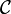${\mathcal C}$ be the set of all Cauchy sequences of rational numbers.

1. Prove that${\mathcal C}$ is a ring if taken with the operations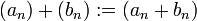$(a_n)+(b_n):=(a_n+b_n)$ and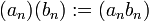$(a_n)(b_n):=(a_nb_n)$.
2. What is the zero element of${\mathcal C}$? What is its unity? Is it a field?
3. Let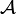${\mathcal A}$ be the set of all sequences of rational numbers that converge to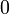$0$. Prove that${\mathcal A}$ is an ideal in${\mathcal C}$.
4. (Hard!) Show that${\mathcal A}$ is a maximal ideal in${\mathcal C}$
5. Can you identify the quotient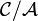${\mathcal C}/{\mathcal A}$ as a ring (a field, by the previous part) you have seen before?
6. Why did I bother asking you this question? In other words, in what sense is this question"useful"?

### Due Date

This assignment is due in class on Wednesday January 24, 2007.